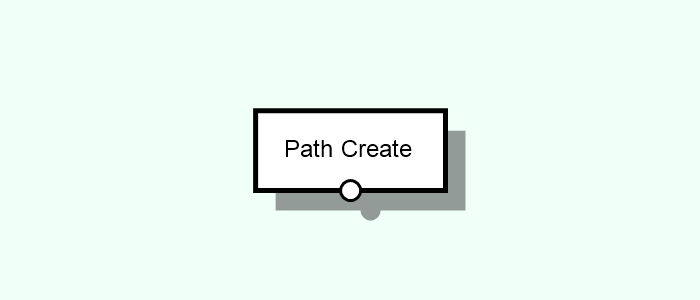# Path Create

Creates Paths of primitive patterns (grid, voronoi) or from other Entity types.Tags: Path, From Mesh, From Points, Grid, Single, Voronoi

## Parameters​

Type [Select]
Type of path pattern/method to create.
From Mesh [Compound] [Adds ports 1]
Creates a path from the given mesh edges.
From Points [Compound] [Adds ports 2]
Creates a path from a set of given points.
Method [Select]
Method to connect the points into the path.
Cross [Compound]
Connects all pairs of points.
Delaunay [Compound]
Connects all points into a delaunay triangle mesh.
Sequence [Compound]
Connects all points in a sequence, in the same order as received in the input.
Voronoi [Compound]
Creates a voronoi pattern from the given points.
Grid [Compound]
Creates a path network with a grid shape.
Number of Columns [Int]
Number of points in the X direction.
Number of Rows [Int]
Number of points in the Y direction.
Column Width [Int]
Spacing between column points (in X).
Row Height [Int]
Spacing between row points (in Y).
Single [Compound]
Creates a path from a sequence of points.
Points [List]
List of points that define the path.
Point [Vector3D]
Coordinate of the point.
Voronoi [Compound]
Creates a path network with a cellular appearance using the Voronoi algorithm.
Height [Int]
Height (size in Y) of the path network.
Width [Int]
Width (size in X) of the path network.
Spacing [Float]
Average spacing between the path intersections.
Max Offset [Float]
Maximum offset of each path intersection. A value of 0 will result in a grid-like appearance.
Seed [Int]
Seed that defines the randomness of the path.

## Inputs​

This node has no native inputs.

### Parameter Inputs​

•  Input [Single | Mesh]: Mesh whose edges are to be extracted.
•  Input [Collective | Point]: Points from which to create path vertices.

## Outputs​

• Output [Path]: Path created according to the defined parameters and/or inputs.